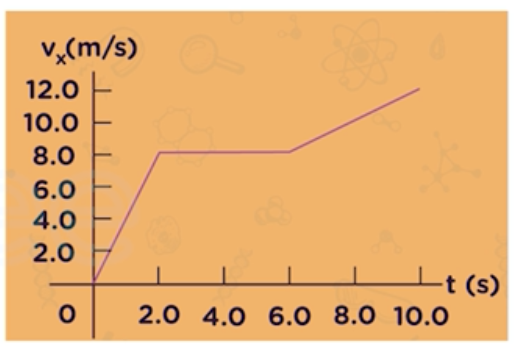Need Help?

Subscribe to Physics 1

###### \${selected_topic_name}
• Notes

$\begin{array}{l}{\text { A } 68.5-\mathrm{kg} \text { skater moving initially at } 2.4 \mathrm{m} / \mathrm{s} \text { on rough horizontal ice comes to rest uniformly }} \\ {\text { in } 3.52 \text { s due to friction from the ice. What force does friction exert on the skater? }}\end{array}$

$m=68.5 \mathrm{kg}$

$V_{{0}_{x}}=2.4 \mathrm{m} / \mathrm{s}$

$V_{x}=Zero \quad 3.52S$

$V_x=V_{{0}_ x}+a_{x} t \rightarrow 0-2.4=a_{x} t$

$\rightarrow a_{x}=\frac{0-2.4}{3.52}=-0.682 \mathrm{m} / \mathrm{s}^{2}$

$f_{x}=m a_{x}=68.5 *(-0.682)=-46.7 N$

$\begin{array}{l}{\text { A box rests on a frozen pond, which serves as a frictionless horizontal surface. If a fisherman }} \\ {\text { applies a horizontal force with magnitude } 48.0 \mathrm{N} \text { to the box and produces an acceleration of }} \\ {\text { magnitude } 3 \mathrm{m} / \mathrm{s}^{\wedge} 2 \text { . What is the mass of the box? }}\end{array}$

$\sum \vec{F}_{x}=m \vec{a}_{x} \longrightarrow m=\frac{\sum \vec{ F}_{x}}{\vec{a}_{x}}=\frac{48}{3}$

$m=16 \mathrm{kg}$

$\begin{array}{l}{\text { A crate with mass } 32.5 \text { initially at rest on a warehouse floor is acted on by a net horizontal force of } 140 \mathrm{N} \text { . }} \\ {\text { (a) What acceleration is produced? }} \\ {\text { (b) How far does the crate travel in } 10.0 \text { s? }} \\ {\text { (c) What is its speed at the end of } 10.0 \mathrm{s} \text { ? }}\end{array}$$m=32.5 \mathrm{kg}$

$V_{0 x}=0$

$F_{x}=140 \mathrm{N}$

$a \ ??$

$x - x_{0} \ ??$

$\sum\vec{F}=m \vec{a}$

$\vec{F}_{x}=m \vec{a}_{x}$

$a_{x}=\frac{F_{x}}{m}=\frac{32.5}{32.5}=4.31 \mathrm{m} / \mathrm{s}^{2}$

$x-x_{0}=V_{0x} F+\frac{1}{2} a_{x} t^{2}$

$x-x_{0}=\frac{1}{2} a_{x} t^{2}=\frac{1}{2}(4.31)(10)^{2}=215 \mathrm{m}$

$\begin{array}{l}{V_{x}=V_{0 x}+a_{x}{t}=(4.31)(10)=43.1 \mathrm{m} / \mathrm{s}}\end{array}$

$\begin{array}{l}{\text { A } 2.75-\mathrm{kg} \text { cat moves in a straight line (the } \mathrm{x} \text { -axis). The Figure shows a graph of the } \mathrm{x} \text { -component of }} \\ {\text { this cat's velocity as a function of time. }} \\ {\text { (a) Find the maximum net force on this cat. When does this force occur? }} \\ {\text { (b) When is the net force on the cat equal to zero? }} \\ {\text { (c) What is the net force at time } 8.5 \mathrm{s} \text { ? }}\end{array}$

* $t=0 \rightarrow t=2 s, \ V=8 \mathrm{m} / \mathrm{s}$

$a_{x}=\frac{8}{2}=4 \mathrm{m} / \mathrm{s}^{2}$

* $t=2 \rightarrow t=6 s, V=8 \mathrm{m} / \mathrm{s}$

$a_{x}=0$

* $t=6 \longrightarrow t=10 \mathrm{s}, \quad 8 \rightarrow 12 \mathrm{m} / \mathrm{s}$

$a_{x}=\frac{12-8}{10-6}=\frac{4}{4}=1m/ s^{2}$

$F_{x}=m a_ x$

$F_{x{1}}=m a_{x1}=2.75 * 4=11 \mathrm{N}$

$F_{x{2}}=ma_{x2}=2.75 * 0=0 \mathrm{N}$

$F_{x{3}}=m a_{x3}=2.75 * 1=2.75 N$

(a) $F_{x}=11 N \quad, \quad t=0 \rightarrow t=2 s$

(b) $F_{x}=0 N \quad t=2 s \rightarrow t=6 s$

(c) $\text { Between } t=6s \rightarrow \mathrm{t}=10 \mathrm{s}, \quad \sum F_{x}=2.75 \mathrm{N}$